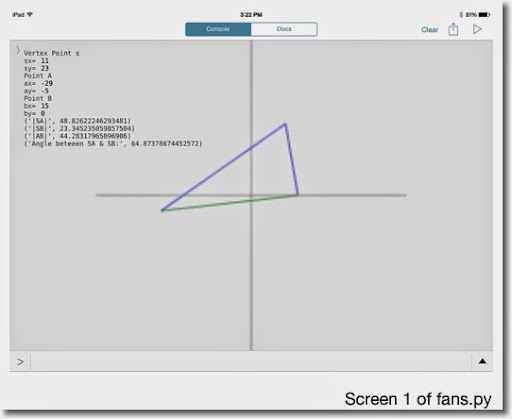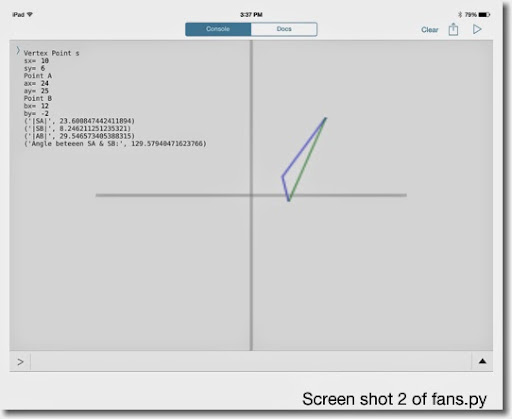## Friday, July 25, 2014

### Pythonista 2.7: Triangle: Lengths of the side and angle given three points

Pythonista Program: fans.py
Version 2.7

Draws a triangle given three points: S, A, and B with coordinates (sx, sy), (ax, ay), and (bx, by), respectively. Lines formed are SA, SB, and AB. This program finds the lengths of SA, SB, and AB, calculates the angle between SA and SB, and draws the triangle.

Note, the modules math and canvas are used. I think the math module is universal but the canvas module seems to exclusive to Pythonista. Not 100% sure. So if you don't have the canvas module, you can omit the second part of the script out. You will just have text.

Example (see screen shot 2):
S = (10,6)
A = (24,25)
B = (12, -2)

Lengths:
SA = 23.600847442411894
SB = 8.246211251235321
AB = 29.546573405388315
Angle = 129.57940471623766°

Scrpit: fans.py

import math
# find distances and angle
# vertex
print('Vertex Point s')
sx=float(input('sx= '))
sy=float(input('sy= '))
# outer points
print('Point A')
ax=float(input('ax= '))
ay=float(input('ay= '))
print('Point B')
bx=float(input('bx= '))
by=float(input('by= '))
# calculate side lengths sa, sb, and ab
# using the hypot function
sa=math.hypot(sx-ax,sy-ay)
sb=math.hypot(sx-bx,sy-by)
ab=math.hypot(ax-bx,ay-by)
print('|SA|', sa)
print('|SB|', sb)
print('|AB|', ab)
# calcuate the angle between sa and sb
ang=math.acos((sa**2+sb**2-ab**2)/(2.*sa*sb))
ang=math.degrees(ang)
print('Angle beteeen SA & SB:', ang)

# drawing lines - Pythonista
import canvas
# set canvas of 100 x 100 to allow room
canvas.set_size(100,100)
# use pixel (50,50) as the origin
# set stroke line
canvas.set_line_width(.25)
# draw lines
# lines sa and sb are blue
canvas.set_stroke_color(0,0,1)
canvas.draw_line(sx+50,sy+50,ax+50,ay+50)
canvas.draw_line(sx+50,sy+50,bx+50,by+50)
# line ab is dark green
canvas.set_stroke_color(0,0.5,0)
canvas.draw_line(ax+50,ay+50,bx+50,by+50)
# draw axis - gray
canvas.set_stroke_color(0.5,0.5,0.5)
canvas.draw_line(0,50,100,50)
canvas.draw_line(50,0,50,100)Kind of a little intro to Pythonista, at least for me.

Eddie

This blog is property of Edward Shore. 2014